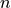# `neighbours` – Ops for working with images in convolutional nets¶

## Functions¶

`aesara.tensor.nnet.neighbours.``images2neibs`(ten4, neib_shape, neib_step=None, mode='valid')[source]

Function `images2neibs` allows to apply a sliding window operation to a tensor containing images or other two-dimensional objects. The sliding window operation loops over points in input data and stores a rectangular neighbourhood of each point. It is possible to assign a step of selecting patches (parameter `neib_step`).

Parameters: ten4 (A 4d tensor-like) – A 4-dimensional tensor which represents a list of lists of images. It should have shape (list 1 dim, list 2 dim, row, col). The first two dimensions can be useful to store different channels and batches. neib_shape (A 1d tensor-like of 2 values) – A tuple containing two values: height and width of the neighbourhood. It should have shape (r,c) where r is the height of the neighborhood in rows and c is the width of the neighborhood in columns. neib_step (A 1d tensor-like of 2 values) – (dr,dc) where dr is the number of rows to skip between patch and dc is the number of columns. The parameter should be a tuple of two elements: number of rows and number of columns to skip each iteration. Basically, when the step is 1, the neighbourhood of every first element is taken and every possible rectangular subset is returned. By default it is equal to `neib_shape` in other words, the patches are disjoint. When the step is greater than `neib_shape`, some elements are omitted. When None, this is the same as neib_shape (patch are disjoint). mode ({'valid', 'ignore_borders', 'wrap_centered', 'half'}) – `valid`Requires an input that is a multiple of the pooling factor (in each direction). `half`Equivalent to ‘valid’ if we pre-pad with zeros the input on each side by (neib_shape//2, neib_shape//2) `full`Equivalent to ‘valid’ if we pre-pad with zeros the input on each side by (neib_shape - 1, neib_shape - 1) `ignore_borders`Same as valid, but will ignore the borders if the shape(s) of the input is not a multiple of the pooling factor(s). `wrap_centered`?? TODO comment Reshapes the input as a 2D tensor where each row is an pooling example. Pseudo-code of the output: ```idx = 0 for i in range(list 1 dim): for j in range(list 2 dim): for k in : for l in : output[idx,:] = flattened version of ten4[i,j,l:l+r,k:k+c] idx += 1 ``` Note The operation isn’t necessarily implemented internally with these for loops, they’re just the easiest way to describe the output pattern. object

Notes

Note

Currently the step size should be chosen in the way that the corresponding dimension(width or height) is equal tofor some.

Examples

```# Defining variables
images = aesara.tensor.type.tensor4('images')
neibs = images2neibs(images, neib_shape=(5, 5))

# Constructing aesara function
window_function = aesara.function([images], neibs)

# Input tensor (one image 10x10)
im_val = np.arange(100.).reshape((1, 1, 10, 10))

# Function application
neibs_val = window_function(im_val)
```

Note

The underlying code will construct a 2D tensor of disjoint patches 5x5. The output has shape 4x25.

`aesara.tensor.nnet.neighbours.``neibs2images`(neibs, neib_shape, original_shape, mode='valid')[source]

Function `neibs2images` performs the inverse operation of `images2neibs`. It inputs the output of `images2neibs` and reconstructs its input.

Parameters: neibs (2d tensor) – Like the one obtained by `images2neibs`. neib_shape – `neib_shape` that was used in `images2neibs`. original_shape – Original shape of the 4d tensor given to `images2neibs` Reconstructs the input of `images2neibs`, a 4d tensor of shape `original_shape`. object

Notes

Currently, the function doesn’t support tensors created with `neib_step` different from default value. This means that it may be impossible to compute the gradient of a variable gained by `images2neibs` w.r.t. its inputs in this case, because it uses `images2neibs` for gradient computation.

Examples

Example, which uses a tensor gained in example for `images2neibs`:

```im_new = neibs2images(neibs, (5, 5), im_val.shape)
# Aesara function definition
inv_window = aesara.function([neibs], im_new)
# Function application
im_new_val = inv_window(neibs_val)
```

Note

The code will output the initial image array.